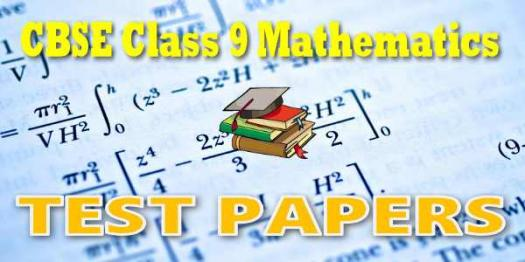Approved & Edited by ProProfs Editorial Team
At ProProfs Quizzes, our dedicated in-house team of experts takes pride in their work. With a sharp eye for detail, they meticulously review each quiz. This ensures that every quiz, taken by over 100 million users, meets our standards of accuracy, clarity, and engagement.
| Written by Tim
T
Tim
Community Contributor
Quizzes Created: 55 | Total Attempts: 19,971
Questions: 10 | Attempts: 283SettingsHow well do you know about angles from the 9th grade? Take this quick and easy 9th grade angles test to find out. We want to see your supposed skils

• 1.

### What divides a figure into two congruent parts?

• A.

Dissect

• B.

Divide

• C.

Bisect

• D.

Circumference

C. Bisect
Explanation
Bisect is the correct answer because when a figure is bisected, it is divided into two congruent parts. This means that both parts are exactly the same in shape and size. Dissect means to cut apart into pieces, which does not necessarily result in congruent parts. Divide can also result in unequal parts. Circumference refers to the distance around a circle and does not involve dividing a figure into congruent parts.

Rate this question:

• 2.

### The largest prefix in the SI system is?

• A.

Deka

• B.

Mili

• C.

Centi

• D.

Kilo

D. Kilo
Explanation
The largest prefix in the SI system is "kilo". This prefix represents a factor of 1000, meaning that any unit of measurement can be multiplied by 1000 when using this prefix. For example, kilogram represents 1000 grams, and kilometer represents 1000 meters. This makes "kilo" the largest prefix in the SI system, as it represents the largest factor of multiplication.

Rate this question:

• 3.

### The parallel slope to: 2x - 3y = 30 is?

• A.

2/3

• B.

-3/2

• C.

2/-3

• D.

3

A. 2/3
Explanation
The given equation is in the form of Ax + By = C, where A = 2, B = -3, and C = 30. To find the parallel slope, we can rearrange the equation to solve for y: -3y = -2x + 30, then divide both sides by -3 to get y = (2/3)x - 10. The coefficient of x, which is 2/3, represents the slope of the line. Therefore, the parallel slope to 2x - 3y = 30 is 2/3.

Rate this question:

• 4.

### An angle bisector is a line that cuts into how many parts?

• A.

5

• B.

2

• C.

3

• D.

4

B. 2
Explanation
An angle bisector is a line that cuts an angle into two equal parts. Therefore, the correct answer is 2.

Rate this question:

• 5.

### The SI unit of mass is?

• A.

Liter

• B.

Meter

• C.

Pound

• D.

Gram

D. Gram
Explanation
The SI unit of mass is the gram. The gram is a unit of measurement for mass in the metric system. It is equal to one thousandth of a kilogram. The gram is commonly used to measure the mass of small objects or quantities of substances.

Rate this question:

• 6.

### Congruent angles are angles that have the same measure and?

• A.

Width

• B.

Shape

• C.

Size

• D.

Length

C. Size
Explanation
Congruent angles are angles that have the same measure and size. The term "size" refers to the magnitude or extent of something. In the context of angles, size refers to the degree of rotation or the amount of turn between the two angles. When two angles have the same measure, it means that they have the same size, indicating that they cover the same amount of rotation or turn. Therefore, "size" is the appropriate term to describe the characteristic of congruent angles.

Rate this question:

• 7.

### A reflex angle is?

• A.

>180˚

• B.

>180˚

• C.

• D.

360˚

B. >180˚
Explanation
A reflex angle is an angle that measures greater than 180˚ and less than 360˚. It is formed by the extension of one of the sides of the angle beyond a straight line. In this case, the answer given is ">180˚", indicating that a reflex angle is any angle that is greater than 180˚.

Rate this question:

• 8.

### What is the slope of a horizontal line?

• A.

1

• B.

0

• C.

-1

• D.

2

B. 0
Explanation
A horizontal line has a slope of 0 because it is parallel to the x-axis and does not have any vertical change. The slope of a line represents the ratio of the vertical change to the horizontal change between any two points on the line. In the case of a horizontal line, the vertical change is 0, resulting in a slope of 0.

Rate this question:

• 9.

### A liter measures the volume of?

• A.

An Angle

• B.

Matter

• C.

Gas

• D.

A Liquid

D. A Liquid
Explanation
A liter is a unit of measurement used to measure the volume of a liquid. It is commonly used to measure the amount of liquid in containers such as bottles, cups, or tanks. The other options, such as an angle, matter, or gas, are not measured in liters.

Rate this question:

• 10.

### When lines intersect, they form what?

• A.

A Right Angle

• B.

An Acute Angle

• C.

A Straight Angle

• D.

An Obtuse AngleBack to top